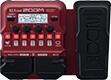# Muse patch - hysteria, psycho

Discussion in 'Zoom B1/B1X Four' started by Sammoore, Feb 23, 2022.

1. Muse patch - hysteria, psychoDevice: Zoom B1X Four
Firmware: 1.10

Name on device: HYSTERIA
Optimized for: Guitar Amp

Effects chain:syn for hysteria

Effect: "ZNR" (Dynamics), active - "yes"
"Detect" = GTRIN
"Depth" = 100
"Threshold" = 40
"Decay" = 0

Effect: "BlackOpt" (Dynamics), active - "yes"
"Comp" = 20
"Lo" = 50
"Hi" = 60
"Volume" = 75

Effect: "Squeak" (Overdrive / Distortion), active - "yes"
"Gain" = 30
"FLTR" = 40
"Volume" = 40
"DryMx" = 50

Effect: "AG 750" (Amp simulator), active - "yes"
"Bass" = 40
"Middle" = 40
"Treble" = 60
"Gain" = 35
"Bright" = On
"Deep" = Off
"Volume" = 70

Effect: "Z-Syn" (Sfx), active - "no"
"FREQ" = 5
"Range" = 15
"Decay" = 100
"RESO" = 15
"Wave" = 1
"Tone" = 5
"BAL" = 1
"VOL" = 70
"Frequency" = 0
"Range" = 0
"Decay" = 0
"Resonance" = 0
"Wave" = SAW
"Tone" = 0
"Balance" = 0
"Volume" = 0

Patch Volume: 100